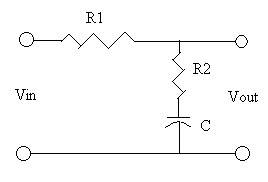# Circuits: Bode Plot Help

• Engineering

## Homework Statement

sketch a bode plot of frequency versus voltage gain (in decibels) for the following circuit:## Homework Equations

Voltage divider: $$V_X = V_S \frac{Z_X}{Z_T}$$
Voltage gain: $$A_V = \frac{V_O}{V_I}$$
log(A)+log(B) = log(A*B)

## The Attempt at a Solution

This is the most complex one i've done up to date, which really just says I'm trying to get a grasp on how to do it. I did all my work following a similar example I've seen done (it's the only example with 3 elements i've seen, thus far)

Step 1: Write voltage gain equation:

$$A_V = \frac{R_2 + \frac{1}{jwc}}{R_1 + R_2 + \frac{1}{jwc}}$$

Step 2: Get into "standard form" (1 divided by 1 plus something according to my prof.)

I factored out a 1/jwC out of top and bottom, then split the numerator out of the fraction:

$$(1 + jwCR_2)*(\frac{1}{1+jwC(R_1 + R_2)}$$

These two factors will be my "A" and "B", which I will solve find the bode plots for individually then add them together to get the overall bode plot. log(A) + log(B) = log(A*B)

step 3:

This is where I get confused when doing these. My professor always takes the jwC term (and anything connected to it), sets it equal to one, and solves omega, now calling it the "break point", then plugs this back into the equation to get a "frequency ratio". It's usually labeled $$W_C$$ but in this case there would be a $$W_1$$ and $$W_2$$; one for each factor. I know how to get one for the 2nd term (B)

For the 2nd term:

$$W_2C(R_1 + R_2) = 1$$
$$\frac{1}{W_2} = C(R_1 + R_2)$$

which I can substitute back into the equation to give me:

$$\frac{1}{1+ \frac{jW}{W_2}}$$

As for the 1st term, I'm not sure what to set equal to one. I tried just setting the $$jWCR_2$$ term equal to one, and in the end I got a +20dB at higher frequencies for the total response...and this seems wrong since all the elements are passive. In the example I've seen, there was only a jWCR2 term without the plus one, and it's throwing me off